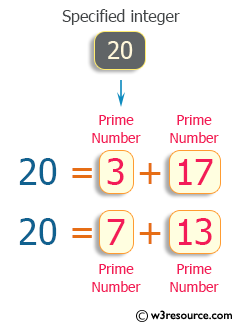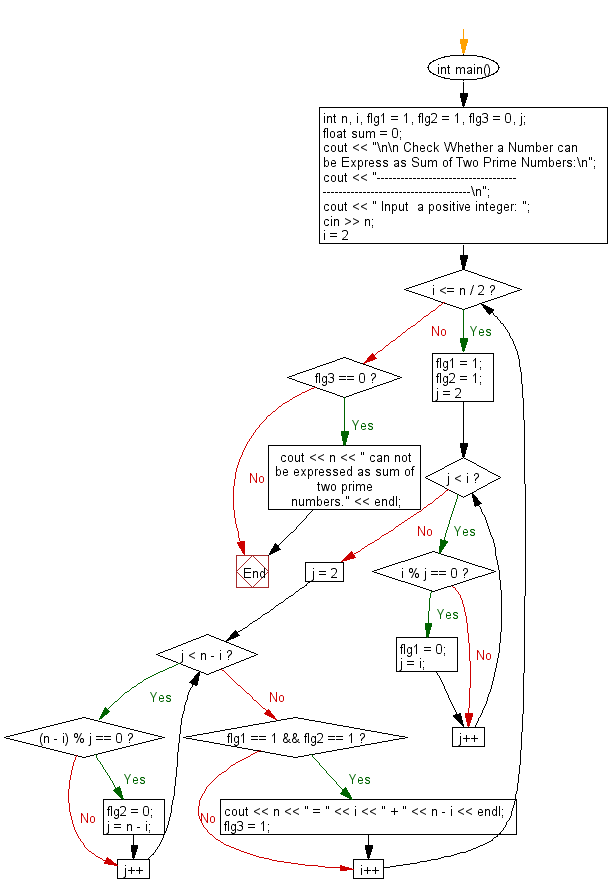﻿ C++ : Check if a number can be Express as Sum of two Primes# C++ Exercises: Check Whether a Number can be Express as Sum of Two Prime Numbers

## C++ For Loop: Exercise-33 with Solution

Write a program in C++ to Check Whether a Number can be Express as Sum of Two Prime Numbers.

Pictorial Presentation:Sample Solution:-

C++ Code :

``````#include <iostream>
using namespace std;

int main()
{
int n, i, flg1 = 1, flg2 = 1, flg3 = 0, j;
float sum = 0;
cout << "\n\n Check Whether a Number can be Express as Sum of Two Prime Numbers:\n";
cout << "------------------------------------------------------------------------\n";
cout << " Input  a positive integer: ";
cin >> n;
for (i = 2; i <= n / 2; i++)
{
/*---------- check for prime---------------*/
flg1 = 1;
flg2 = 1;
for (j = 2; j < i; j++)
{
if (i % j == 0)
{
flg1 = 0;
j = i;
}
}
for (j = 2; j < n - i; j++)
{
if ((n - i) % j == 0)
{
flg2 = 0;
j = n - i;
}
}
if (flg1 == 1 && flg2 == 1)
{
cout << n << " = " << i << " + " << n - i << endl;
flg3 = 1;
}
}
if (flg3 == 0)
{
cout << n << " can not be expressed as sum of two prime numbers." << endl;
}
}
``````

Sample Output:

``` Check Whether a Number can be Express as Sum of Two Prime Numbers:
------------------------------------------------------------------------
Input  a positive integer: 20
20 = 3 + 17
20 = 7 + 13
```

Flowchart:C++ Code Editor:

Contribute your code and comments through Disqus.

What is the difficulty level of this exercise?

﻿